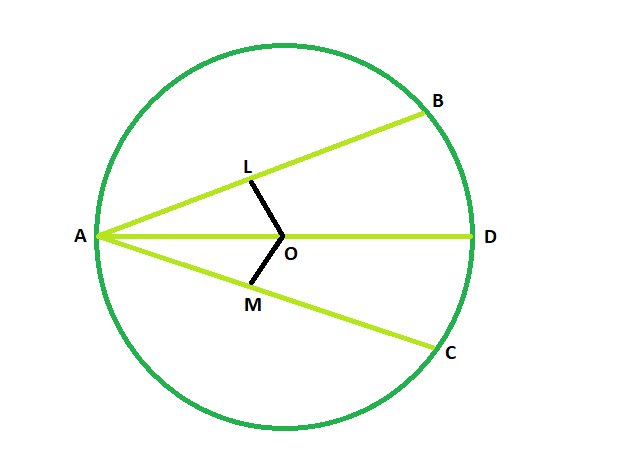Open in App
Not now

# Length of the chord the circle if length of the another chord which is equally inclined through the diameter is given

• Last Updated : 30 May, 2022

Given are two chords which are equally inclined through the diameter of the circle. Length of one chord is given. The task is to find the length of another chord.
Examples:

```Input: z = 48
Output: 48 degrees

Input: z = 93
Output: 93 degrees```Approach

• Let AB and AC be the two chords of the circle having the center at O.
• now, in the figure we see,
OL is perpendicular to AB and OM is perpendicular to AC
• in triangle OLA and triangle OMA
angle OLA = angle OMA = 90 degrees
angle OAL = angle OAM(as the chords are inclined equally through the diameter)
OA = OA(common side)
• so triangle OLA and triangle OMA are congruent to each other.
• So, OL = OM
• and we know, equal chords are equidistant from the center, so Length of AB and AC will be same.

If two chords are equally inclined through the diameter of the same circle, then they are of equal length.

Below is the implementation of the above approach:

## C++

 `// C++ program to find``// the length of the chord the circle``// if length of the another chord``// which is equally inclined``// through the diameter is given` `#include ``using` `namespace` `std;` `void` `lengchord(``int` `z)``{``    ``cout << ``"The length is "``         ``<< z << endl;``}` `// Driver code``int` `main()``{``    ``int` `z = 48;``    ``lengchord(z);``    ``return` `0;``}`

## Java

 `// Java program to find``// the length of the chord the circle``// if length of the another chord``// which is equally inclined``// through the diameter is given``import` `java.io.*;` `class` `GFG``{``    ` `static` `void` `lengchord(``int` `z)``{``    ``System.out.println (``"The length is "``+ z );``}` `// Driver code``public` `static` `void` `main (String[] args)``{` `    ``int` `z = ``48``;``    ``lengchord(z);``}``}` `// The code has been contributed by ajit.`

## Python3

 `# Python3 program to find``# the length of the chord of the circle``# if length of the other chord``# which is equally inclined``# through the diameter is given``def` `lengchord(z):``    ``print``(``"The length is "``, end ``=` `"");``    ``print``(z);` `# Driver code``z ``=` `48``;``lengchord(z);` `# This code is contributed``# by Princi Singh`

## C#

 `// C# program to find``// the length of the chord the circle``// if length of the another chord``// which is equally inclined``// through the diameter is given``using` `System;` `class` `GFG``{``        ` `static` `void` `lengchord(``int` `z)``{``    ``Console.WriteLine(``"The length is "``+ z );``}` `// Driver code``static` `public` `void` `Main ()``{``        ` `    ``int` `z = 48;``    ``lengchord(z);``}``}` `// The code has been contributed by Tushil`

## Javascript

 ``

Output:

`The length is 48`

Time Complexity: O(1)

Auxiliary Space: O(1)

My Personal Notes arrow_drop_up